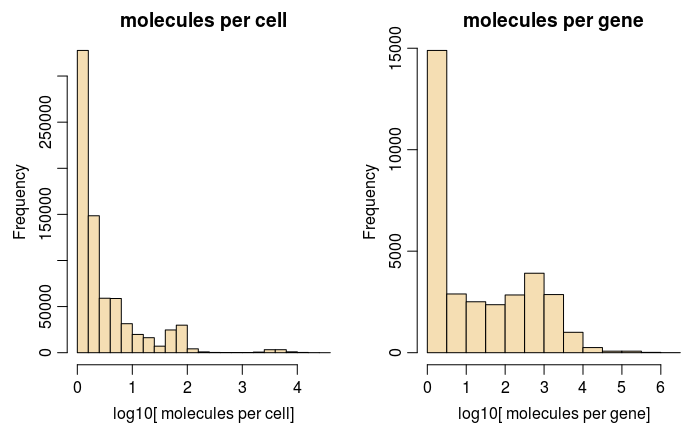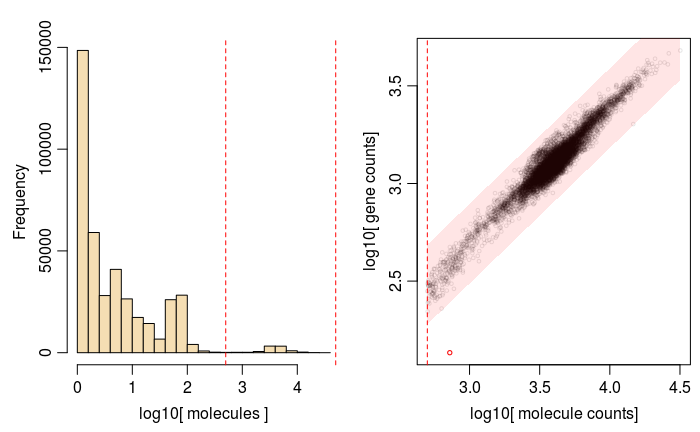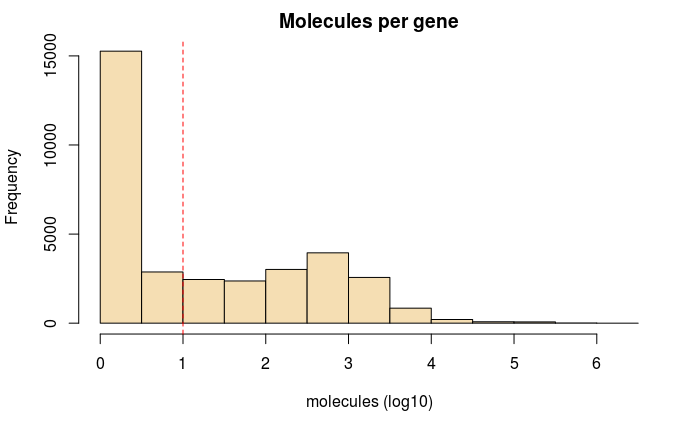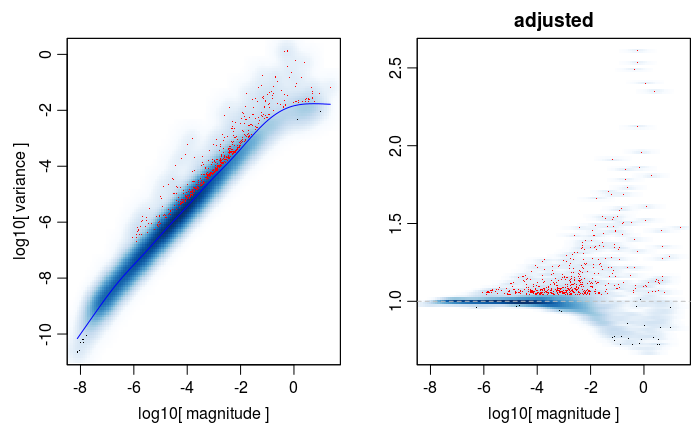This is a quick walkthrough covering key PAGODA2 usage patterns to date.

We will analyze an 8k PBMC dataset from 10x as an example (starting with unfiltered read count matrices).

Try executing this chunk by clicking the Run button within the chunk or by placing your cursor inside it and pressing Cmd+Shift+Enter.

``library(pagoda2)``

Now let’s load the 10x matrices:

``cd <- read.10x.matrices(list(PBMC8K='pbmc8k/raw_gene_bc_matrices/GRCh38'))[]``
``reading 1 dataset(s) . done``
``str(cd)``
``````Formal class 'dgCMatrix' [package "Matrix"] with 6 slots
..@ i       : int [1:17171166] 1614 21625 110 153 231 484 548 550 633 673 ...
..@ p       : int [1:737281] 0 2 2 2 116 120 120 125 126 126 ...
..@ Dim     : int [1:2] 33694 737280
..@ Dimnames:List of 2
.. ..\$ : chr [1:33694] "RP11-34P13.3" "FAM138A" "OR4F5" "RP11-34P13.7" ...
.. ..\$ : chr [1:737280] "PBMC8K_AAACCTGAGAAACCAT-1" "PBMC8K_AAACCTGAGAAACCGC-1" "PBMC8K_AAACCTGAGAAACCTA-1" "PBMC8K_AAACCTGAGAAACGAG-1" ...
..@ x       : num [1:17171166] 1 1 1 1 1 2 1 1 1 1 ...
..@ factors : list()``````

Look at the summary counts across genes and across cells:

``````par(mfrow=c(1,2), mar = c(3.5,3.5,2.0,0.5), mgp = c(2,0.65,0), cex = 1.0)
hist(log10(colSums(cd)+1),main='molecules per cell',col='wheat',xlab='log10[ molecules per cell]')
hist(log10(rowSums(cd)+1),main='molecules per gene',col='wheat',xlab='log10[ molecules per gene]')``````This is unfiltered data, so most of the barcodes are empty/background. Let’s require at least 500 molecules per cell, and use our quick umi-gene relationship function to filter the cells.

``counts <- gene.vs.molecule.cell.filter(cd,min.cell.size=500)````str(counts)``
``````Formal class 'dgCMatrix' [package "Matrix"] with 6 slots
..@ i       : int [1:11965872] 33 45 59 153 335 375 408 440 484 495 ...
..@ p       : int [1:8787] 0 871 1677 2993 3891 5417 6912 8165 9598 11230 ...
..@ Dim     : int [1:2] 33694 8786
..@ Dimnames:List of 2
.. ..\$ : chr [1:33694] "RP11-34P13.3" "FAM138A" "OR4F5" "RP11-34P13.7" ...
.. ..\$ : chr [1:8786] "PBMC8K_AAACCTGAGCATCATC-1" "PBMC8K_AAACCTGAGCTAACTC-1" "PBMC8K_AAACCTGAGCTAGTGG-1" "PBMC8K_AAACCTGCACATTAGC-1" ...
..@ x       : num [1:11965872] 1 1 1 5 1 1 1 1 18 1 ...
..@ factors : list()``````

We can also looka at the number of molecules per gene, and omit low-expressed genes to save computational time:

``````hist(log10(rowSums(counts)+1),main='Molecules per gene',xlab='molecules (log10)',col='wheat')
abline(v=1,lty=2,col=2)````````````counts <- counts[rowSums(counts)>=10,]
str(counts)``````
``````Formal class 'dgCMatrix' [package "Matrix"] with 6 slots
..@ i       : int [1:11947057] 11 17 30 84 174 192 207 222 247 257 ...
..@ p       : int [1:8787] 0 869 1675 2991 3889 5412 6907 8158 9590 11219 ...
..@ Dim     : int [1:2] 15556 8786
..@ Dimnames:List of 2
.. ..\$ : chr [1:15556] "RP11-34P13.7" "FO538757.2" "AP006222.2" "RP4-669L17.10" ...
.. ..\$ : chr [1:8786] "PBMC8K_AAACCTGAGCATCATC-1" "PBMC8K_AAACCTGAGCTAACTC-1" "PBMC8K_AAACCTGAGCTAGTGG-1" "PBMC8K_AAACCTGCACATTAGC-1" ...
..@ x       : num [1:11947057] 1 1 1 5 1 1 1 1 18 1 ...
..@ factors : list()``````

Now we have a clean/lean count matrix and are ready to start analysis. First we’ll create pagoda2 object that will maintain all of the results. It will also provide handles for running all operations on the data. Use of log scale is recommended, as it makes some statistics smoother.

``r <- Pagoda2\$new(counts,log.scale=TRUE)``
``````Error in setCountMatrix(x, min.cells.per.gene = min.cells.per.gene, trim = trim,  :
duplicate gene names are not allowed - please reduce``````

Yes, we need to make gene names unique:

``````rownames(counts) <- make.unique(rownames(counts))
r <- Pagoda2\$new(counts,log.scale=TRUE, n.cores=20)``````
``8786 cells, 13004 genes; normalizing ... using plain model winsorizing ... log scale ... done.``

Note, that above we’ve also specified the default number of processing cores that will be used to speed up some of the analysis steps below. Please match it to your system.

Next, we’ll adjust the variance, to normalize the extent to which genes with (very) different expression magnitudes will contribute to the downstream anlaysis:

``r\$adjustVariance(plot=T,gam.k=10)``
``calculating variance fit ... using gam ``
``````Loading required package: mgcv
This is mgcv 1.8-18. For overview type 'help("mgcv-package")'.``````
``538 overdispersed genes ... 538 persisting ... done.``There are many alternative ways of proceeding with the downstream analysis. Below we’ll use the simplest, default scenario, where we first reduce the dataset dimensions by running PCA, and then move into k-nearest neighbor graph space for clustering and visualization calculations. First, the PCA reduction:

``r\$calculatePcaReduction(nPcs=50,n.odgenes=3e3)``
``Loading required package: irlba``

The next few steps will make kNN graph, find clusters and generate a quick largeVis embedding to visualize the subpopulations:

``r\$makeKnnGraph(k=40,type='PCA',center=T,distance='cosine');``
``````Loading required package: igraph

Attaching package: ‘igraph’

The following objects are masked from ‘package:stats’:

decompose, spectrum

The following object is masked from ‘package:base’:

union``````
``````creating space of type angular done
``````r\$getKnnClusters(method=infomap.community,type='PCA')
``Estimating embeddings.``
``````0%   10   20   30   40   50   60   70   80   90   100%
``r\$plotEmbedding(type='PCA',show.legend=F,mark.clusters=T,min.group.size=50,shuffle.colors=F,mark.cluster.cex=1,alpha=0.1,main='clusters (largeVis)')``